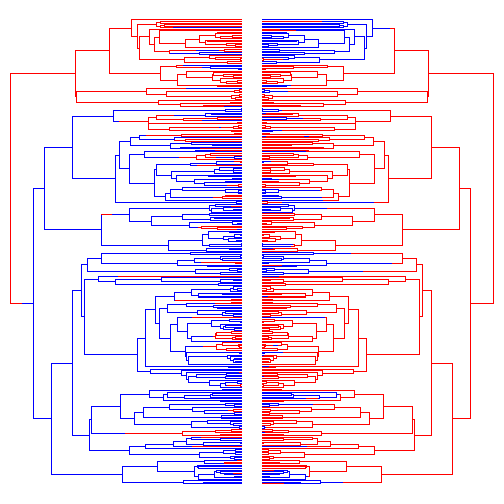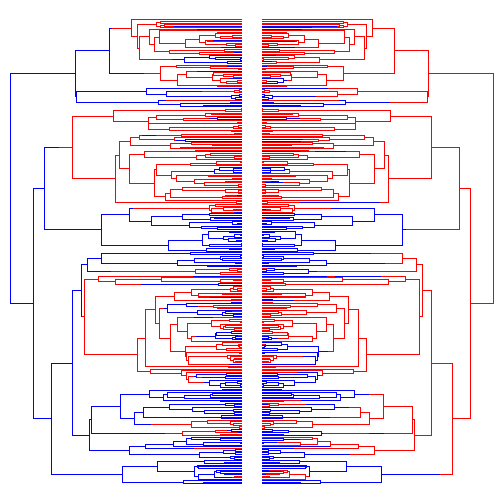## Wednesday, December 3, 2014

### R function for Pagel's 1994 correlation method

I just posted some code to fit the Pagel (1994) method for detecting “correlated” evolution of two binary traits. I say correlated because it might be more appropriate to call this a dependence - i.e., the rate of character 2 depends on 1 & vice versa.

This is actually quite simple to do because the test is merely a test of, for a two character combination written as `` (meaning state `0` for the first character & state `1` for the second), the rates of transition from `->` equal `->`; `->` equal `->`; etc. For this we can just paste our two input characters together, fit both a model in which the dependence exists and one in which it does not, and then compare them. The dependence model has twice as many transition rates as the independence model, so this model will have (for two binary traits) 4 more parameters.

Note that I accomplished this by borrowing `ace` in the ape package or `fitDiscrete` in the geiger package to actually fit the models. All my code really does is pre- & post-process the data & results respectively!

Here's a demo:

``````## first load packages & source code
library(phytools)
library(geiger)
source("fitPagel.R")
.check.pkg<-phytools:::.check.pkg
## now let's simulate some uncorrelated data
tree<-pbtree(n=300,scale=1)
Q<-matrix(c(-1,1,1,-1),2,2)
rownames(Q)<-colnames(Q)<-letters[1:2]
tt1<-sim.history(tree,Q)
``````
``````## Done simulation(s).
``````
``````tt2<-sim.history(tree,Q)
``````
``````## Done simulation(s).
``````
``````## these are uncorrelated, see:
par(mfrow=c(1,2))
plotSimmap(tt1,setNames(c("blue","red"),letters[1:2]),ftype="off",lwd=1)
plotSimmap(tt2,setNames(c("blue","red"),letters[1:2]),ftype="off",lwd=1,direction="leftwards")
````````````x<-tt1\$states
y<-tt2\$states
fit.ape<-fitPagel(tree,x,y)
fit.ape
``````
``````##
##   Pagel's binary character correlation test:
##
## Indepedent model rate matrix:
##         a|a     a|b     b|a     b|b
## a|a -1.9712  1.0896  0.8816  0.0000
## a|b  0.9774 -1.8589  0.0000  0.8816
## b|a  0.7531  0.0000 -1.8426  1.0896
## b|b  0.0000  0.7531  0.9774 -1.7304
##
## Dependent model rate matrix:
##         a|a     a|b     b|a     b|b
## a|a -1.5606  0.8578  0.7028  0.0000
## a|b  0.9261 -1.8634  0.0000  0.9373
## b|a  0.3652  0.0000 -1.5764  1.2112
## b|b  0.0000  1.2754  1.0567 -2.3321
##
## Model fit:
##             log-likelihood
## independent         -238.7
## dependent           -237.5
##
## Hypothesis test result:
##   likelihood-ratio:  2.390211
##   p-value:  0.6643971
``````
``````fit.geiger<-fitPagel(tree,x,y,method="fitDiscrete")
``````
``````## Warning: Parameter estimates appear at bounds:
##  q14
##  q23
##  q32
##  q41
## Warning: Parameter estimates appear at bounds:
##  q14
##  q23
##  q32
##  q41
``````
``````fit.geiger
``````
``````##
##   Pagel's binary character correlation test:
##
## Indepedent model rate matrix:
##         a|a     a|b     b|a     b|b
## a|a -1.8847  0.9942  0.8906  0.0000
## a|b  0.9699 -1.8605  0.0000  0.8906
## b|a  0.7112  0.0000 -1.7053  0.9942
## b|b  0.0000  0.7112  0.9699 -1.6811
##
## Dependent model rate matrix:
##         a|a     a|b     b|a     b|b
## a|a -1.5000  0.8192  0.6809  0.0000
## a|b  0.8987 -1.8596  0.0000  0.9609
## b|a  0.3704  0.0000 -1.4820  1.1116
## b|b  0.0000  1.1176  1.1075 -2.2251
##
## Model fit:
##             log-likelihood
## independent         -239.3
## dependent           -238.3
##
## Hypothesis test result:
##   likelihood-ratio:  2.096727
##   p-value:  0.7179738
``````

OK, now let's try correlated data. To do that I will have to build a big transition matrix myself for simulation!

``````Q<-matrix(c(0,0.5,0.5,0,2,0,0,2,2,0,0,2,0,0.5,0.5,0),4,4,byrow=TRUE)
rownames(Q)<-colnames(Q)<-c("aa","ab","ba","bb")
diag(Q)<--rowSums(Q)
tt<-sim.history(tree,t(Q))
``````
``````## Note - the rate of substitution from i->j should be given by Q[j,i].
## Done simulation(s).
``````
``````tt1<-mergeMappedStates(tt,c("aa","ab"),"a")
tt1<-mergeMappedStates(tt1,c("ba","bb"),"b")
tt2<-mergeMappedStates(tt,c("aa","ba"),"a")
tt2<-mergeMappedStates(tt2,c("ab","bb"),"b")
## these data are correlated, see:
par(mfrow=c(1,2))
plotSimmap(tt1,setNames(c("blue","red"),letters[1:2]),ftype="off",lwd=1)
plotSimmap(tt2,setNames(c("blue","red"),letters[1:2]),ftype="off",lwd=1,direction="leftwards")
````````````x<-getStates(tt1,"tips")
y<-getStates(tt2,"tips")
fit.ape<-fitPagel(tree,x,y)
fit.ape
``````
``````##
##   Pagel's binary character correlation test:
##
## Indepedent model rate matrix:
##         a|a     a|b     b|a     b|b
## a|a -1.4325  0.9831  0.4493  0.0000
## a|b  0.9593 -1.4086  0.0000  0.4493
## b|a  1.0401  0.0000 -2.0233  0.9831
## b|b  0.0000  1.0401  0.9593 -1.9994
##
## Dependent model rate matrix:
##        a|a     a|b     b|a    b|b
## a|a -1.043  0.8539  0.1893  0.000
## a|b  5.543 -7.9019  0.0000  2.359
## b|a  1.202  0.0000 -4.4114  3.209
## b|b  0.000  1.0849  0.4054 -1.490
##
## Model fit:
##             log-likelihood
## independent         -241.1
## dependent           -207.8
##
## Hypothesis test result:
##   likelihood-ratio:  66.63346
##   p-value:  1.164753e-13
``````
``````fit.geiger<-fitPagel(tree,x,y,method="fitDiscrete")
``````
``````## Warning: Parameter estimates appear at bounds:
##  q14
##  q23
##  q32
##  q41
## Warning: Parameter estimates appear at bounds:
##  q14
##  q23
##  q32
##  q41
``````
``````fit.geiger
``````
``````##
##   Pagel's binary character correlation test:
##
## Indepedent model rate matrix:
##         a|a    a|b     b|a     b|b
## a|a -1.3892  1.006  0.3832  0.0000
## a|b  0.9236 -1.307  0.0000  0.3832
## b|a  1.0639  0.000 -2.0699  1.0061
## b|b  0.0000  1.064  0.9236 -1.9875
##
## Dependent model rate matrix:
##         a|a     a|b        b|a    b|b
## a|a -0.8951  0.8951  1.799e-16  0.000
## a|b  5.4502 -7.7907  0.000e+00  2.340
## b|a  1.9288  0.0000 -3.958e+00  2.029
## b|b  0.0000  1.0086  4.105e-01 -1.419
##
## Model fit:
##             log-likelihood
## independent         -241.9
## dependent           -208.8
##
## Hypothesis test result:
##   likelihood-ratio:  66.16744
##   p-value:  1.460391e-13
``````

That's it, I guess. This is brand new so I have no idea how it works but feedback is welcome. I'm also well aware of the recent critique of this method by Maddision & Fitzjohn.

1.great post! I'm new to the field and this really helps getting some orientation

2.Thanks for this.

I am still unsure about one thing though: How does one interpret the rates in the Independent & Dependent model rate matrices? What are the units? Do the cells contain the expected number of substitutions per tree? or per tree height? ... Or is it more complicated than that?

Thank you!

1.Hi Caitlin.

The rate Q(a|a -> b|a), for instance, is the instantaneous rate of transition from a->b in character 1, given that character 2 is in state a. In the independent model, the rate of change in 1 cannot be influenced by character 2, consequently Q(a|a->b|a)==Q(a|b->b|b) & so on.

The rates become probabilities after matrix exponentiation of Q*t for elapsed time t; however another intuitive interpretation is that for a lineage starting in state a with Q(a->b)=2, the expected number of changes in a unit of time is 2.

Hope this helps. Liam

3.Hello again,

I believe I understand your first point. The example you gave would correspond to the fact that in an independent Q rate matrix, the contents of the cells [1,3] and [2,4] will be equal, correct?

With regard to your second point, though, I confess to remaining a little unsure of myself. I think I agree that the rates are more easily interpreted as numbers of substitutions per unit time. If you have a moment, could I please try to run a small example past you to see if I am understanding you correctly?

Suppose I have an independent rate matrix, iQ, and I want to use the rates estimated therein to simulate data on a given tree. The rate Q(b|a->a|a) (also Q(b|b->a|b)) in my iQ is 0.79, which is the expected number of changes to occur in a unit time.

My tree is not a timed tree, but I can use max(nodeHeights(tree)) to get its height, which is 1.4. The sum of the branch lengths in my tree is 9.5. Am I correct in thinking that there are 9.5/1.4=6.79 “units of time” in my tree? If so, would the expected value of the number of substitutions to occur for this variable, throughout the tree, be 0.79*6.79=5.36?

Finally, if I wanted to simulate data for this variable on this tree using this rate estimate, what would be the recommended procedure for getting an actual value for the number of substitutions to occur in a given simulation (for example, should I round down to 5, or draw a number from a Poisson distribution with parameter 5.36)?

Thanks sincerely,
Caitlin.

4.Hi,

Edit--sorry, I just realised it probably makes more sense for 1 to be one unit of time in my tree... If the sum of the branch lengths is 9.5, are there 9.5 "units of time" in my tree?

Best,
Caitlin.

1.Yes, the amount of time in your tree for things to occur is the sum of the edge lengths. Exactly.

5.Hi Liam,

I hope you are well

I was wondering how this could be apply to estimate significant negative correlation between traits. For my correlation between two traits, my likelihood-ratio ratio is negative -16.19662, and the p-value is 1, which for me looks very suspicious given that this likelihood ratio is pretty high. Therefore, I was wondering that maybe it is not testing for negative correlation.

Thank you so much in advance!

Wendy

1.Hi Wendy.

If your LR is -16.2 that's bad. It means that your dependent model has a worse likelihood than your independent model - even though the former has the latter as a special case! What it must mean is that your dependent model failed to optimize.

As a first step, you could change the value of method - which sets the function that is used internally for optimization. (That is, if you had method="fitMk" you can change it to method="fitDiscrete", and vice versa.)

If that doesn't work, please save & email me your R workspace & the command that creates this problem via email and I'll look into it.

- Liam

6.Hi Liam,

Thank you so much for your prompt response.

I changed the method to "fitDiscrete" and now I'm getting a reasonable non-negative LR. However, I was also wondering if I could use different models in the fitPagel function, beyond the ER and the ARD (something like GTR). I tried to specify some models on a matrix and then pass it to the fitPagel, and also inspecting the function trying to chance the iQ matrix but it just says that the model is invalid, and uses ARD as default. Another related question, is that I calculated weigthed AIC for the three models (ER,ARD, and SYM(ER)), for the two individual traits that I'm using, but what if they have different models as the best fit?

Also, just to be sure, if I'm getting that the correlation is significant (LR=14.49, p-value<0.001) how do I know if the traits are negatively or positively correlated? (I suspect they are negatively correlated because a naive, not phylogenetically corrected Pearson correlation coefficient is negative).

Finally, I was wondering if there's any way to account for incomplete sampling, because I have phenotypic data for more species than I have in the phylogeny, and the genera are monophyletic, and I would like to include this data.

Sorry for the many and very basic questions but I'm just very new to this...

Thank you so much in advance,

Wendy

7.Dear Liam,

I follow your blog with great enthusiasm. Thank you for providing such valuable information.

I performed the tests as explained and received statistically significant difference, but I am not really sure how to interpret the matrix. I understand that x and y are correlated, but I would like to see how the exact changes of the variable states are correlated. However, to give you an example, I compare morphological traits i.e. spines - presence/absence; spots - presence/absence. I want to see if the appearance of spines means losing the spots and vice versa. Do I need a post hoc test, or the matrix is enough for such conclusion?

Thank you very much in advance!

Cheers,
1.